# 4. 蒙特卡洛方法

（相对于从部分收益率学习的方法，将在之后考虑）。

## 4.1 蒙特卡洛预测

Frist-visit MC prediction, for estimating V ≈ v π ​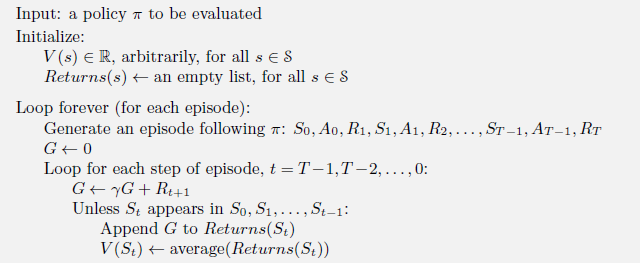### 例4.1:Blackjack(21点)## 4.3 Monte Carlo 控制为了使蒙特卡罗方法的收敛性得到保证，我们在上面做了两个不太可能的假设。一个是事件有探索的开始，另一个是策略评估可以用无数的事件来完成。为了得到一个实用的算法，我们必须去除这两个假设。

Monte Carlo ES (Exploring Starts), for estimating π ≈ π ∗ ​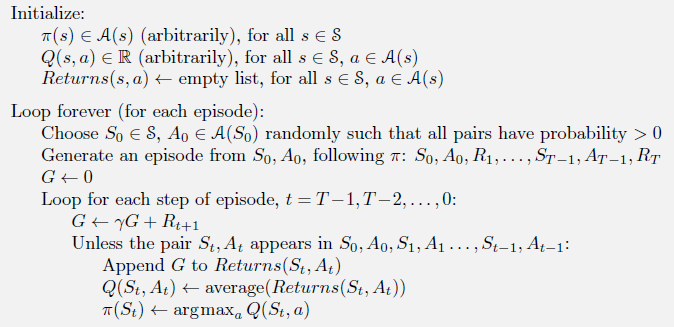### 例4.2: 21点的解法### 4.4 蒙特卡洛控制的无探索启动​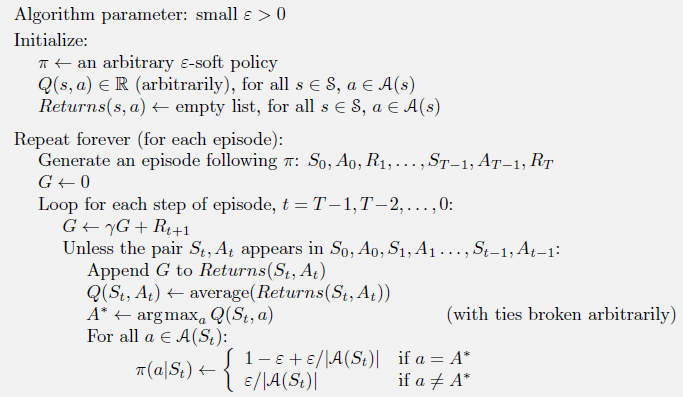\varepsilonε-柔性策略中找到了最佳策略。这种分析与在每个阶段如何确定动作-价值函数无关，但它假定它们是精确计算出来的。现在我们只选择柔性策略中最好的一个，但另

## 4.5 通过重要性采样进行Off-policy预测其中，p为状态转移概率函数。因此，目标和行为策略下轨迹的相对概率(重要采样比率)为## 4.6 增量实现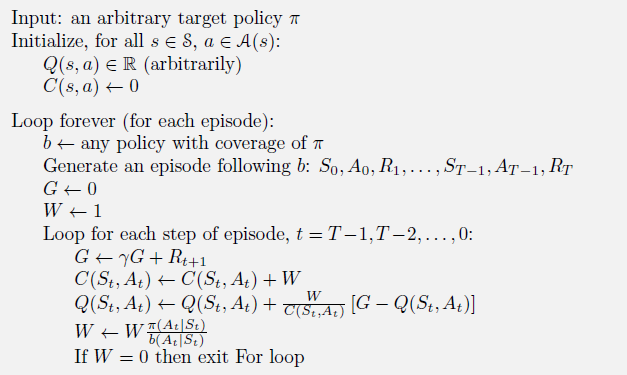## 4.7 Off-policy蒙特卡洛控制

off-policy蒙特卡罗控制方法使用前面两部分中介绍的技术之一。他们在学习和改进目标策略的同时遵循行为策略。这些技术要求行为策略选择目标策略(覆盖率)可能选择的所有操作的概率为非零。为了探索所有的可能性，我们要求行为策略是柔性的(例如，即以非零概率选择所有状态下的所有动作)。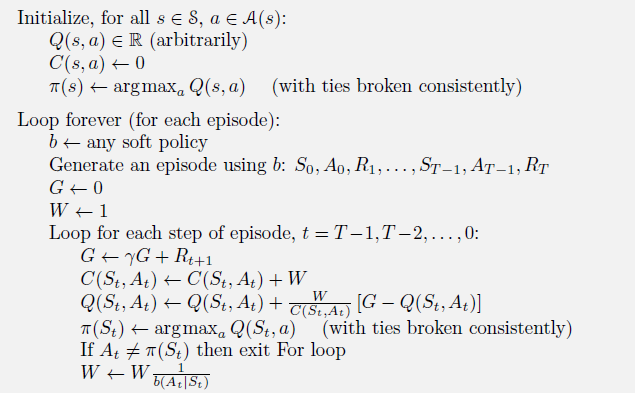## 4.8 案例: 21点游戏

21点的游戏规则是这样的：游戏里有一个玩家（player）和一个庄家（dealer），每个回合的结果可能是玩家获胜、庄家获胜或打成平手。回合开始时，玩家和庄家各有两张牌，

A    1或11
2    2
3    3
…    …
9    9
10, J ,Q, K    10

## 4.8.1 实验环境的使用

Gym库的环境’Blackjack-v0’实现了上述21点游戏。21点游戏环境和Gym库中其他环境的用法大致相同，我们可以用以下语句取得环境对象：

import gym
env = gym.make("Blackjack-v0")

env.reset()

env.step(action)

env.step()函数的参数是动作，它可以是int型数值0或1，其中0表示玩家不再要更多的牌，1表示玩家再要一张牌。env.step()的第0个返回值是观测，它是一个有3个元素的tuple值，其3个元素依次为：

bool型数值，表示在计算玩家点数和的时候，是否有将1张A牌计算为11点。

if __name__ == '__main__':
env = gym.make("Blackjack-v0")
observation = env.reset()
print('观测 = {}'.format(env.player, env.dealer))
while True:
print('玩家 = {}, 庄家 = {}'.format(env.player, env.dealer))
action = np.random.choice(env.action_space.n)
print('动作 = {}'.format(action))
observation, reward, done, _ = env.step(action)
print('观测 = {}, 奖励 = {}, 结束指示 = {}'.format(observation, reward, done))

if done:
break

### 4.8.2 策略评估

21点游戏的交互可以看作一个Markov决策过程。我们将观测略做修改，将观测tuple的最后一个元素改为int值，就可以得到用3个int值表示的状态，见以下代码。

# 从观测到状态
def ob2state(observation):
return(observation, observation, int(observation))

# 回合更新策略评估
def evaluate_action_monte_carlo(env, policy, episode_num=50000):
q = np.zeros_like(policy)
c = np.zeros_like(policy)
for _ in range(episode_num):
# 玩一回合
state_actions = []
observation = env.reset()
while True:
state = ob2state(observation)
action = np.random.choice(env.action_space.n, p=policy[state])
state_actions.append((state, action))
observation, reward, done, _ = env.step(action)
if done:
break
g = reward
for state, action in state_actions:
c[state][action] += 1.
q[state][action] += (g - q[state][action]) / c[state][action]
return q


evaluate_action_monte_carlo()函数，求得其动作价值函数为q 。接着，利用动作价值函数求出了状态价值函数v 。

policy = np.zeros((22, 11, 2, 2))
policy[20:, :, :, 0] = 1  # >20时不再要牌
policy[:20, :, :, 1] = 1  # <20时不再要牌
q = evaluate_action_monte_carlo(env, policy)  # 动作价值
v = (q * policy).sum(axis=-1)

# 绘制最后一维的指标为0或1的3维数组
def plot(data):
fig, axes = plt.subplots(1, 2, figsize=(9, 4))
titles = ['without ace', 'with ace']
have_aces = [0, 1]
extent = [12, 22, 1, 11]
for title, have_ace, axis in zip(titles, have_aces, axes):
dat = data[extent:extent, extent:extent, have_ace].T
axis.imshow(dat, extent=extent, origin='lower')
axis.set_xlabel('player sum')
axis.set_ylabel('dealer showing')
axis.set_title(title)
plt.show()

plot(v)### 4.8.3 on-policy最优策略求解

# 带起始探索的回合更新
# 带起始探索的回合更新
def monte_carlo_with_exploring_start(env, episode_num=500000):
policy = np. zeros((22, 11, 2, 2))
policy[:, :, :, 1] = 1.
q = np.zeros_like(policy)
c = np.zeros_like(policy)
for _ in range(episode_num):
# 随机选择起始状态和起始动作
state = (np.random.randint(12, 22),
np.random.randint(1, 11),
np.random.randint(2))
action = np.random.randint(2)
# 玩一回合
env.reset()
if state:  # 有A
env.player = [1, state - 11]
else:  # 没有A
if state == 21:
env.player = [10, 9, 2]
else:
env.player = [10, state - 10]
env.dealer = state
state_actions = []
while True:
state_actions. append((state, action))
observation, reward, done, _ = env. step(action)
if done:
break  # 回合结束
state = ob2state(observation)
action = np.random.choice(env.action_space.n, p=policy[state])
g = reward  # 回报
for state, action in state_actions:
c[state][action] += 1.
q[state][action] += (g - q[state][action]) / c[state][action]
a = q[state].argmax()
policy[state] = 0.
policy[state][a] = 1.
return policy, q


 # 带起始探索的更新策略
print('带起始探索的更新策略'.center(20, '-'))
policy_wes, q = monte_carlo_with_exploring_start(env)
v = q.max(axis=-1)
plot(policy_wes.argmax(-1))
plot(v)### 4.8.4 off-policy策略评估# 重要性采样策略评估
def evaluate_monte_carlo_importance_resample(env, policy, behavior_policy, episode_num=500000):
q = np.zeros_like(policy)
c = np.zeros_like(policy)
for _ in range(episode_num):
# 用行为策略玩一回合
state_actions = []
observation = env.reset()
while True:
state = ob2state(observation)
action = np.random.choice(env. action_space.n, p=behavior_policy[state])
state_actions.append((state, action))
observation, reward, done, _ = env.step(action)
if done:
break  # 玩好了
g = reward  # 回报
rho = 1.  # 重要性采样比率
for state, action in reversed(state_actions):
c[state][action] += rho
q[state][action] += (rho / c[state][action]*(g - q[state][action]))
rho *= (policy[state][action]/ behavior_policy[state][action])
if rho == 0:
break  # 提前终止
return q

evaluate_action_monte_carlo_importance_resample()函数的用法如下列代码所示。其中的行为策略是π ( a ∣ s ) = 0.5 π(a|s)=0.5π(a∣s)=0.5（s ∈ S , a ∈ A s∈\mathcal{S},a∈\mathcal{A}s∈S,a∈A）。该代码可以生成与on-policy回合更新一致的结果。

policy = np.zeros((22, 11, 2, 2))
policy[20:, :, :, 0] = 1  # >= 20时收手
policy[:20, :, :, 1] = 1  # <20时继续
behavior_policy = np.ones_like(policy) * 0.5
q = evaluate_monte_carlo_importance_resample(env, policy, behavior_policy)
v = (q * policy).sum(axis=-1)
plot(v)



### 4.8.5 off-policy最优策略求解

# 柔性策略重要性采样最优策略求解
def monte_carlo_importance_resample(env, episode_num=500000):
policy = np.zeros((22, 11, 2, 2))
policy[:, :, :, 0] = 1.
behavior_policy = np.ones_like(policy) * 0.5  # 柔性策略
q = np.zeros_like(policy)
c = np.zeros_like(policy)
for _ in range(episode_num):
# 用行为策略玩一回合
state_actions = []
observation = env.reset()
while True:
state = ob2state(observation)
action = np.random.choice(env.action_space.n, p=behavior_policy[state])
state_actions.append((state, action))
observation, reward, done, _= env.step(action)
if done:
break  # 玩好了
g = reward  # 回报
rho = 1.  # 重要性采样比率
for state, action in reversed(state_actions):
c[state][action] += rho
q[state][action] += (rho / c[state][action]*(g - a[state][action]))
# 策略改进
a = q[state].argmax()
policy[state] = 0.
policy[state][a] = 1.
if a != action:  # 提前终止
break
rho /= behavior_policy[state][action]
return policy, q
=

policy, q = monte_carlo_importance_resample(env)
v = q.max(axis=-1)
plot(policy.argmax(-1))
plot(v)

4.8.6 蒙特卡洛方法完整代码

import gym
import numpy as np
import matplotlib.pyplot as plt

# 从观测到状态
def ob2state(observation):
return(observation, observation, int(observation))

# 回合更新策略评估
def evaluate_action_monte_carlo(env, policy, episode_num=50000):
q = np.zeros_like(policy)
c = np.zeros_like(policy)
for _ in range(episode_num):
# 玩一回合
state_actions = []
observation = env.reset()
while True:
state = ob2state(observation)
action = np.random.choice(env.action_space.n, p=policy[state])
state_actions.append((state, action))
observation, reward, done, _ = env.step(action)
if done:
break
g = reward
for state, action in state_actions:
c[state][action] += 1.
q[state][action] += (g - q[state][action]) / c[state][action]
return q

# 绘制最后一维的指标为0或1的3维数组
def plot(data):
fig, axes = plt.subplots(1, 2, figsize=(9, 4))
titles = ['without ace', 'with ace']
have_aces = [0, 1]
extent = [12, 22, 1, 11]
for title, have_ace, axis in zip(titles, have_aces, axes):
dat = data[extent:extent, extent:extent, have_ace].T
axis.imshow(dat, extent=extent, origin='lower')
axis.set_xlabel('player sum')
axis.set_ylabel('dealer showing')
axis.set_title(title)
plt.show()

# 带起始探索的回合更新
def monte_carlo_with_exploring_start(env, episode_num=500000):
policy = np. zeros((22, 11, 2, 2))
policy[:, :, :, 1] = 1.
q = np.zeros_like(policy)
c = np.zeros_like(policy)
for _ in range(episode_num):
# 随机选择起始状态和起始动作
state = (np.random.randint(12, 22),
np.random.randint(1, 11),
np.random.randint(2))
action = np.random.randint(2)
# 玩一回合
env.reset()
if state:  # 有A
env.player = [1, state - 11]
else:  # 没有A
if state == 21:
env.player = [10, 9, 2]
else:
env.player = [10, state - 10]
env.dealer = state
state_actions = []
while True:
state_actions. append((state, action))
observation, reward, done, _ = env. step(action)
if done:
break  # 回合结束
state = ob2state(observation)
action = np.random.choice(env.action_space.n, p=